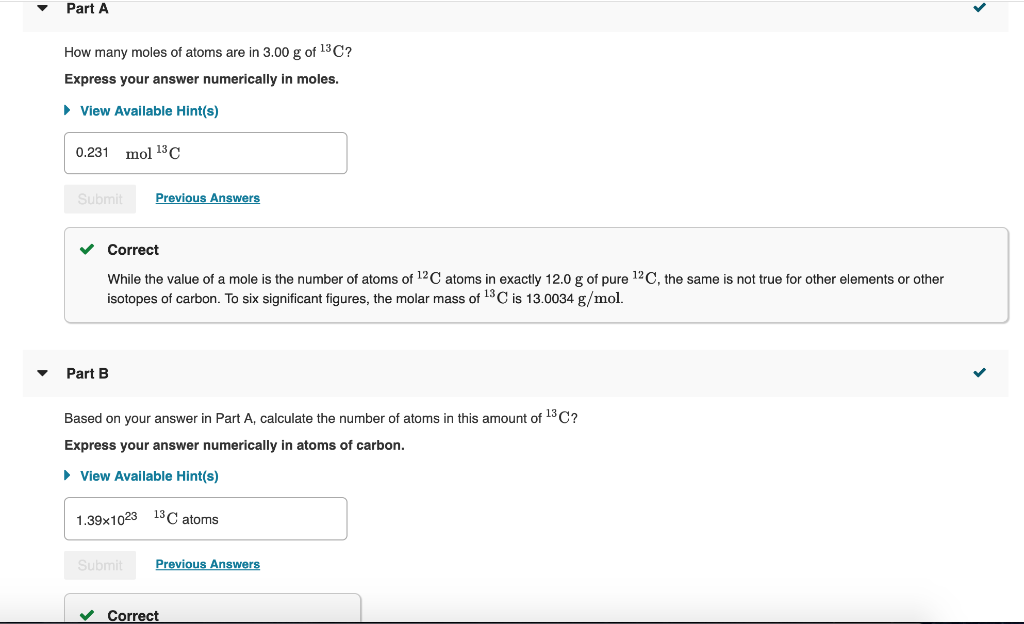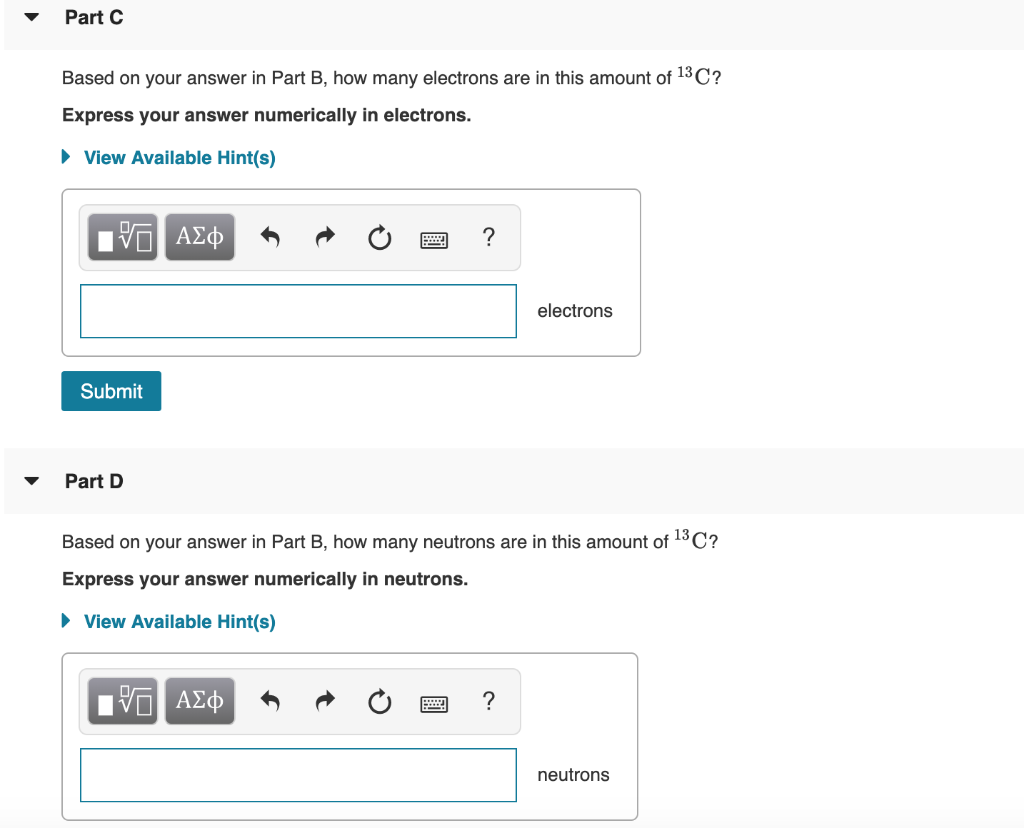# Based on your answer in part b. how many electrons are in this amount of 13c?

Part C. Based on your answer in Part B, how many electrons are
in this amount of 13C? Express your answer numerically in electrons. Part D. Based on your answer in Part B, how many neutrons are in
this amount of 13C? Express your answer numerically in neutrons.Part A How many moles of atoms are in 3.00 g of 13C? Express your answer numerically in moles. View Available Hint(s) 0.231 mol 13C Previous An Correct While the value of a mole is the number of atoms of 12C atoms in exactly 12.0 g of pure 12C, the same is not true for other elements or other isotopes of carbon. To six significant figures, the molar mass of 13 C is 13.0034 g/mol. Part Based on your answer in Part A, calculate the number of atoms in this amount of 13C? Express your answer numerically in atoms of carbon. View Available Hint(s) 1.39x102313C atoms Previous Answers Correct Part C Based on your answer in Part B, how many electrons are in this amount of 13C? Express your answer numerically in electrons. View Available Hint(s) electrons Submit Part D Based on your answer in Part B, how many neutrons are in this amount of 13C? Express your answer numerically in neutrons. View Available Hint(s) neutrons

Atomic number of carbon is 6.
For Neutral atom, Atomic number = No of protons = No of
electrons
No of neutrons = Mass number – atomic number
In case , C-13 . number of protons = 6 and number of electrons =
6 and number of neutrons = 7 .
We can write , 1 C-13 atom contain 6 electrons. Therefore, 1.39
x 10 23 C-13 atoms contain ( 1.39 x 10 23 x 6
= 8.34 x 10 23
ANSWER : Number of electrons in 1.39 x 10 23
C-13 atoms = 8.34 x 10 23
PART D
We can write , 1 C-13 atom contain 7 neutrons. Therefore, 1.39 x
10 23 C-13 atoms contain ( 1.39 x 10 23 x 7 =
9.73 x 10 23
ANSWER : Number of neutrons in 1.39 x 10 23
C-13 atoms = 9.73 x 10 23